Parents and Teachers: Support Ducksters by following us onor.

Kids Math

Simplifying and Reducing Fractions

One of the things you have to do at the end of most fraction problems is to simplify or reduce the fraction. When you reduce a fraction, you don't change the actual value of the fraction, you just write it down in its simplest form.

How do you know if a fraction is fully reduced?

Writing a fraction in its simplest form means that the top and bottom numbers can no longer be divided by the same whole number exactly or evenly (other than the number 1).

For example, the fraction 2/3 is fully reduced. There isn't any whole number, other than 1, that both 2 and 3 can be divided by without having a remainder. Other examples of fully reduced fractions include 7/8, 5/9, and 11/20.

An example of a fraction that isn't fully reduced is 2/4. This is because both 2 and 4 can be divided by 2 to equal the fraction ½. You can see from the picture below that these fractions are the same, but ½ is the simpler of the two fractions and is fully reduced.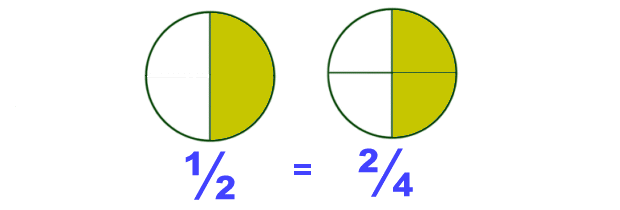Other examples of fractions that can be further reduced include 3/12, 16/20, 8/24.

How to Reduce Fractions

One way to reduce fractions is to find the greatest common factor of the numerator and the denominator. Here are the steps to follow:
• Write down the factors for the numerator and the denominator
• Determine the largest factor that is common between the two
• Divide the numerator and denominator by the greatest common factor
• Write down the reduced fraction
Example:

Reduce the fractionStep 1:

Factors for 8 = 1, 2, 4, 8
Factors for 24 = 1, 2, 3, 4, 6, 8, 12, 24

Step 2:

The greatest common factor is 8

Step 3:

Divide both the numerator and denominator by 8

8 divided by 8 = 1
24 divided by 8 = 3

Step 4:More examples: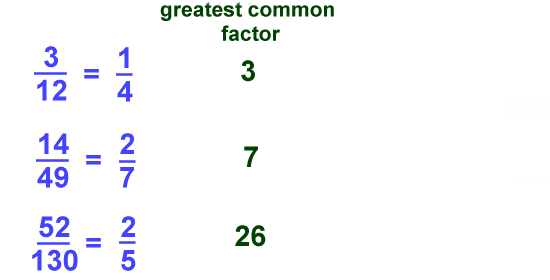Mixed Numbers

Another part of writing the correct answer of a fraction problem can be converting the fraction to a mixed number. This is a number that is part whole number and part fraction. If the numerator is larger than the denominator, then the fraction can be written as a mixed number.

Basic Example: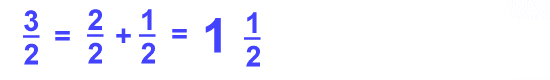As you can see the fraction 3/2 can be written as 1 ½. These numbers are both the same value, but sometimes the answer will be need to be written as a mixed number to be considered fully reduced or simplified.

Converting Improper Fractions to Mixed Numbers

To convert an improper fraction to a mixed number, follow these steps:
• Divide the numerator by the denominator
• Write the result as the whole number
• Write any remainder as the numerator of the fraction
• The denominator stays the same
Example: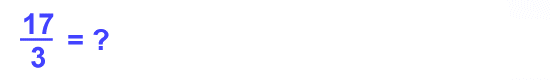Divide the numerator 17 by the denominator 3.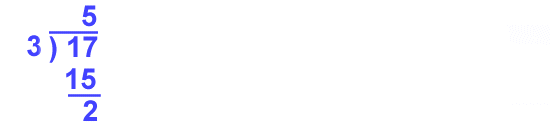You get 5 with the remainder 2. Write out the answer with the 5 as the whole number and the remainder 2 over the original denominator 3.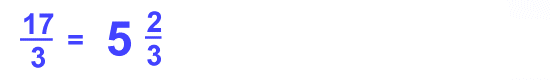Back to Kids Math

Back to Kids StudyFollow us onor